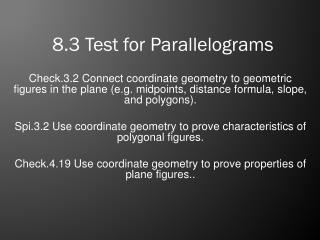DownloadDownload Presentation8.3 Test for Parallelograms

# 8.3 Test for Parallelograms

Download Presentation## 8.3 Test for Parallelograms

- - - - - - - - - - - - - - - - - - - - - - - - - - - E N D - - - - - - - - - - - - - - - - - - - - - - - - - - -
##### Presentation Transcript

1. 8.3 Test for Parallelograms Check.3.2Connect coordinate geometry to geometric figures in the plane (e.g. midpoints, distance formula, slope, and polygons). Spi.3.2 Use coordinate geometry to prove characteristics of polygonal figures. Check.4.19 Use coordinate geometry to prove properties of plane figures..

2. Parallelograms A parallelogram is a quadrilateral with both pairs of opposite sides parallel. A B D C Properties of Parallelogram – Converse Statements Opposite Sides of a parallelogram are congruent Opposite Angles of a parallelogram are congruent Consecutive Angles of a parallelogram are supplementary. Diagonals of a parallelogram bisect each other

3. Proving Parallelograms A B D C If opposite sides of a quadrilateral are parallel, then it is a parallelogram

4. Proving Parallelograms A B D C If opposite sides of a quadrilateral are congruent, then it is a parallelogram

5. Parallelograms A B D C If both pairs of opposite angles of a quadrilateral are congruent then it is a parallelogram

6. Parallelograms A B D C If diagonals of a quadrilateral bisect each other, then then it is a parallelogram

7. Proving Parallelograms A B D C If one pair of opposite sides of a quadrilateral is both parallel and congruent, then it is a parallelogram

8. Proving Parallelograms Given AC & BD If both pairs of opposite angles of a quadrilateral are congruent then it is a parallelogram CPCTC, If opposite sides of a quadrilateral are congruent, then it is a parallelogram

9. Proving Parallelograms If both pairs of opposite angles of a quadrilateral are congruent then it is a parallelogram If opposite sides of a quadrilateral are congruent, then it is a parallelogram

10. Using Properties Find x and y so that the quadrilateral is a parallelogram. If opposite sides of a quadrilateral are congruent, then it is a parallelogram 4y = 6y - 42 6x - 12 = 2x + 36 6x – 2x = 36 + 12 42 = 6y – 4y 4x = 48 42 = 2y x = 12 y = 21

11. Using Properties Find x and y so that the quadrilateral is a parallelogram. If diagonals of a quadrilateral bisect each other, then , then it is a parallelogram x = 5x - 28 2y + 12 = 5y 12 = 3y 28 = 4x y = 4 x=7

12. Using Slope to prove Parallelograms If opposite sides of a quadrilateral are parallel then it is a parallelogram. If two lines are parallel, they have the same slope. AD BC AB DC Both sides have the same slope, therefore they are parallel and it is a parallelogram

13. Using Slope and Distance to prove Parallelograms Is PS  QR and is PS parallel with QR? Since one pair of opposite sides is congruent and parallel, then PQRS is a parallelogram.

14. Summary – Test for Parallelograms • Both pairs of opposite sides are parallel • Both pairs of opposite sides are congruent. • Both pairs of opposite angles are congruent. • Diagonals Bisect each other • A pair of opposite sides are both parallel and congruent. • Practice Assignment • Page 414 10 -24 Even